• +91 9971497814
• info@interviewmaterial.com

# Arithmetic Progressions Ex-5.1 Interview Questions Answers

### Related Subjects

Question 1 : In which of the following situations, does the list of numbers involved make as arithmetic progression and why?

(i) The taxi fare after each km when the fare is ₹ 15 for the first km and ₹ 8 for each additional km.
(ii) The amount of air present in a cylinder when a vacuum pump removes 14 of the air remaining in the cylinder at a time.
(iii) The cost of digging a well after every meter of digging, when it costs ₹ 150 for the first meter and rises by ₹ 50 for each subsequent meter.
(iv) The amount of money in the account every year, when ₹ 10000 is deposited at compound interest at 8% per annum.
Solution-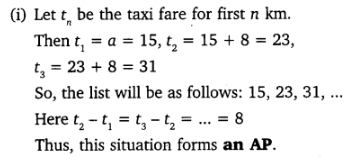Solution-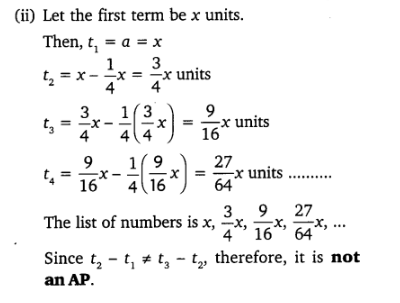Solution-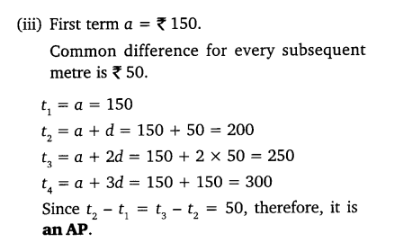Solution-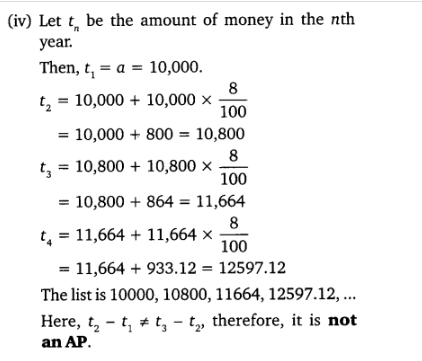Question 2 : Write first four terms of the A.P. when the first term a and the common difference are given as follows:

(i) a = 10, d = 10
(ii) a = -2, d = 0
(iii) a = 4, d = – 3
(iv) a = -1 d = 1/2
(v) a = – 1.25, d = – 0.25

Solution

(i) a = 10, d =10

Let us consider, the Arithmetic Progressionseries be a1, a2, a3, a4, a5 …

a1 = a = 10

a2 = a1+d = 10+10 = 20

a3 = a2+d = 20+10 = 30

a4 = a3+d = 30+10 = 40

a5 = a4+d = 40+10 = 50

And so on…

Therefore, the A.P. series will be 10, 20, 30,40, 50 …

And First four terms of this A.P. will be 10,20, 30, and 40.

Solution

(ii) a = – 2, d =0

Let us consider, the Arithmetic Progressionseries be a1, a2, a3, a4, a5 …

a1 = a = -2

a2 = a1+d = – 2+0 = – 2

a3 = a2+d = – 2+0 = – 2

a4 = a3+d = – 2+0 = – 2

Therefore, the A.P. series will be – 2, – 2, –2, – 2 …

And, First four terms of this A.P. will be –2, – 2, – 2 and – 2.

Solution

(iii) a = 4, d =– 3

Let us consider, the Arithmetic Progressionseries be a1, a2, a3, a4, a5 …

a1 = a = 4

a2 = a1+d = 4-3 = 1

a3 = a2+d = 1-3 = – 2

a4 = a3+d = -2-3 = – 5

Therefore, the A.P. series will be 4, 1, – 2 –5 …

And, first four terms of this A.P. will be 4,1, – 2 and – 5.

Solution

(iv) a = – 1, d =1/2

Let us consider, the Arithmetic Progressionseries be a1, a2, a3, a4, a5 …

a2 = a1+d = -1+1/2 = -1/2

a3 = a2+d = -1/2+1/2 = 0

a4 = a3+d = 0+1/2 = 1/2

Thus, the A.P. series will be-1, -1/2, 0, 1/2

And First four terms of this A.P. will be -1,-1/2, 0 and 1/2.

Solution

(v) a = – 1.25, d =– 0.25

Let us consider, the Arithmetic Progressionseries be a1, a2, a3, a4, a5 …

a1 = a = – 1.25

a2 = a1 + d = –1.25-0.25 = – 1.50

a3 = a2 + d = –1.50-0.25 = – 1.75

a4 = a3 + d = –1.75-0.25 = – 2.00

Therefore, the A.P series will be 1.25, –1.50, – 1.75, – 2.00 ……..

And first four terms of this A.P. will be –1.25, – 1.50, – 1.75 and – 2.00.

Question 3 : For the following APs, write the first term and the common difference:

(i) 3, 1, -1, -3, ……
(ii) -5, -1, 3, 7, ……
(iii) 13 , 53 , 93, 133 , ……..
(iv) 0.6, 1.7, 2.8, 3.9, …….
Solution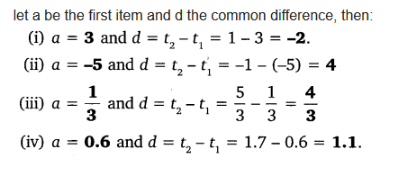Question 4 : Which of the following are APs? If they form an A.P. find the common difference d and write three more terms.

(i) 2, 4, 8, 16 …
(ii) 2, 5/2, 3, 7/2 ….
(iii) -1.2, -3.2, -5.2, -7.2 …
(iv) -10, – 6, – 2, 2 …
(v) 3, 3 + √2, 3 + 2√2, 3 + 3√2
(vi) 0.2, 0.22, 0.222, 0.2222 ….
(vii) 0, – 4, – 8, – 12 …
(viii) -1/2, -1/2, -1/2, -1/2 ….
(ix) 1, 3, 9, 27 …
(x) a, 2a, 3a, 4a …
(xi) aa2a3a4 …
(xii) √2, √8, √18, √32 …
(xiii) √3, √6, √9, √12 …
(xiv) 12, 32, 52, 72 …
(xv) 12, 52, 72, 73 …

Solution

(i) Given to us,

2, 4, 8, 16 …

Here, the common difference is;

a2 – a1 = 4 – 2 = 2

a3 – a2 = 8 – 4 = 4

a4 – a3 = 16 – 8 = 8

Since, an+1 – aorthe common difference is not the same every time.

Therefore, the given series are not forming anA.P.

Solution

(ii) Given, 2, 5/2,3, 7/2 ….

Here,

a2 – a1 = 5/2-2 = 1/2

a3 – a2 = 3-5/2 = 1/2

a4 – a3 = 7/2-3 = 1/2

Since, an+1 – an orthe common difference is same every time.

Therefore, d = 1/2 andthe given series are in A.P.

The next three terms are;

a5 = 7/2+1/2 = 4

a6 = 4 +1/2 = 9/2

a7 = 9/2 +1/2 = 5

Solution

(iii) Given,-1.2, – 3.2, -5.2, -7.2 …

Here,

a2 – a1 = (-3.2)-(-1.2) = -2

a3 – a2 = (-5.2)-(-3.2) = -2

a4 – a3 = (-7.2)-(-5.2) = -2

Since, an+1 – an orcommon difference is same every time.

Therefore, d = -2 and thegiven series are in A.P.

Hence, next three terms are;

a5 = – 7.2-2 = -9.2

a6 = – 9.2-2 = – 11.2

a7 = – 11.2-2 = – 13.2

Solution

(iv) Given, -10, –6, – 2, 2 …

Here, the terms and their difference are;

a2 – a1 = (-6)-(-10) = 4

a3 – a2 = (-2)-(-6) = 4

a4 – a3 = (2 -(-2) = 4

Since, an+1 – an orthe common difference is same every time.

Therefore, d = 4 and thegiven numbers are in A.P.

Hence, next three terms are;

a5 = 2+4 = 6

a6 = 6+4 = 10

a7 = 10+4 = 14

Solution

(v) Given, 3, 3+√2,3+2√2, 3+3√2

Here,

a2 – a1 = 3+√2-3 = √2

a3 – a2 = (3+2√2)-(3+√2) = √2

a4 – a3 = (3+3√2) – (3+2√2) = √2

Since, an+1 – an orthe common difference is same every time.

Therefore, d = √2 andthe given series forms a A.P.

Hence, next three terms are;

a5 = (3+√2) +√2 = 3+4√2

a6 = (3+4√2)+√2 = 3+5√2

a7 = (3+5√2)+√2 = 3+6√2

Solution

(vi) 0.2, 0.22,0.222, 0.2222 ….

Here,

a2 – a1 = 0.22-0.2 = 0.02

a3 – a2 = 0.222-0.22 = 0.002

a4 – a3 = 0.2222-0.222 =0.0002

Since, an+1 – an orthe common difference is not same every time.

Therefore, and the given series doesn’t formsa A.P.

Solution

(vii) 0, -4,-8, -12 …

Here,

a2 – a1 = (-4)-0 = -4

a3 – a2 = (-8)-(-4) = -4

a4 – a3 = (-12)-(-8) = -4

Since, an+1 – an orthe common difference is same every time.

Therefore, d = -4 andthe given series forms a A.P.

Hence, next three terms are;

a5 = -12-4 = -16

a6 = -16-4 = -20

a7 = -20-4 = -24

Solution

(viii) -1/2, -1/2,-1/2, -1/2 ….

Here,

a2 – a1 = (-1/2) – (-1/2) = 0

a3 – a2 = (-1/2) – (-1/2) = 0

a4 – a3 = (-1/2) – (-1/2) = 0

Since, an+1 – an orthe common difference is same every time.

Therefore, d = 0 and thegiven series forms a A.P.

Hence, next three terms are;

a5 = (-1/2)-0 = -1/2

a6 = (-1/2)-0 = -1/2

a7 = (-1/2)-0 = -1/2

Solution

(ix) 1, 3, 9, 27 …

Here,

a2 – a1 = 3-1 = 2

a3 – a2 = 9-3 = 6

a4 – a3 = 27-9 = 18

Since, an+1 – an orthe common difference is not same every time.

Therefore, and the given series doesn’t form aA.P.

Solution

(x) a, 2a,3a, 4a …

Here,

a2 – a1 = 2aa

a3 – a2 = 3a-2a = a

a4 – a3 = 4a-3a = a

Since, an+1 – an orthe common difference is same every time.

Therefore, d = a andthe given series forms a A.P.

Hence, next three terms are;

a5 = 4a+a = 5a

a6 = 5a+a = 6a

a7 = 6a+a = 7a

Solution

(xi) aa2a3a4 …

Here,

a2 – a1 = a2a =a(a-1)

a3 – a2 = a aa2(a-1)

a4 – a3 = a4 – aa3(a-1)

Since, an+1 – an orthe common difference is not same every time.

Therefore, the given series doesn’t forms aA.P.

Solution

(xii) √2, √8, √18,√32 …

Here,

a2 – a1 = √8-√2  =2√2-√2 = √2

a3 – a2 = √18-√8 =3√2-2√2 = √2

a4 – a3 = 4√2-3√2 = √2

Since, an+1 – an orthe common difference is same every time.

Therefore, d = √2 andthe given series forms a A.P.

Hence, next three terms are;

a5 = √32+√2 = 4√2+√2 = 5√2 = √50

a6 = 5√2+√2 = 6√2 = √72

a7 = 6√2+√2 = 7√2 = √98

Solution

(xiii) √3, √6,√9, √12 …

Here,

a2 – a1 = √6-√3 =√3×√2-√3 = √3(√2-1)

a3 – a2 = √9-√6 =3-√6 = √3(√3-√2)

a4 – a3 = √12 – √9 =2√3 – √3×√3 = √3(2-√3)

Since, an+1 – an orthe common difference is not same every time.

Therefore, the given series doesn’t form aA.P.

Solution

(xiv) 12,32, 52, 72 …

Or, 1, 9, 25, 49 …..

Here,

a2 − a1 = 9−1 = 8

a3 − a= 25−9 = 16

a4 − a3 = 49−25 = 24

Since, an+1 – an orthe common difference is not same every time.

Therefore, the given series doesn’t form aA.P.

Solution

(xv) 12,52, 72, 73 …

Or 1, 25, 49, 73 …

Here,

a2 − a1 = 25−1 = 24

a3 − a= 49−25 = 24

a4 − a3 = 73−49 = 24

Since, an+1 – an orthe common difference is same every time.

Therefore, d = 24 andthe given series forms a A.P.

Hence, next three terms are;

a5 = 73+24 = 97

a6 = 97+24 = 121

a= 121+24 = 145

Todays Deals### Arithmetic Progressions Ex-5.1 Contributorskrishan

Name:
Email:

# Latest News# 9000 interview questions in different categories